Скачать презентацию Forecasting FX Rates Fundamental and Technical Models

e1ccbed63f54d611380e3a643b552001.ppt

• Количество слайдов: 29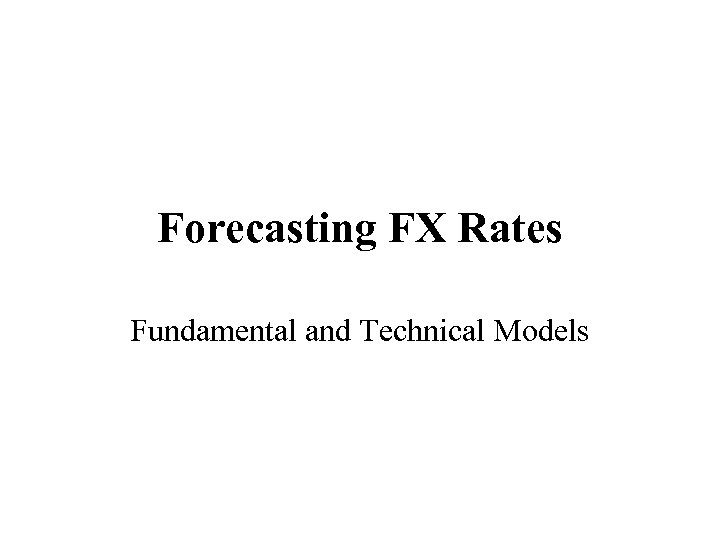Forecasting FX Rates Fundamental and Technical Models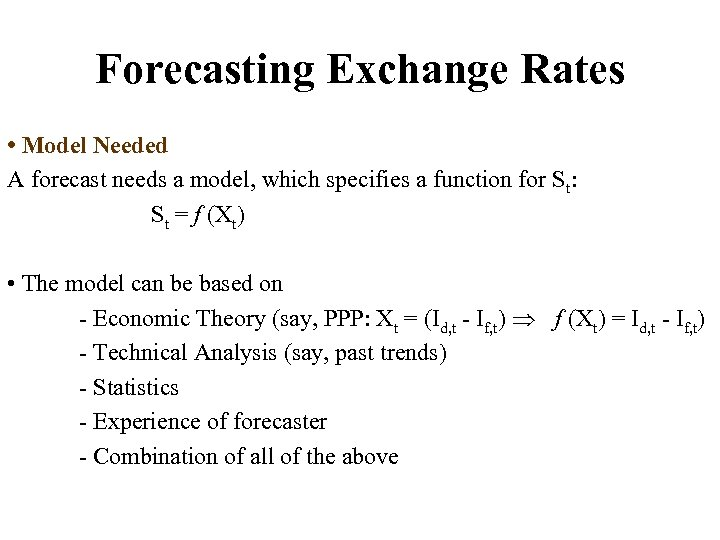Forecasting Exchange Rates • Model Needed A forecast needs a model, which specifies a function for St: St = f (Xt) • The model can be based on - Economic Theory (say, PPP: Xt = (Id, t - If, t) f (Xt) = Id, t - If, t) - Technical Analysis (say, past trends) - Statistics - Experience of forecaster - Combination of all of the above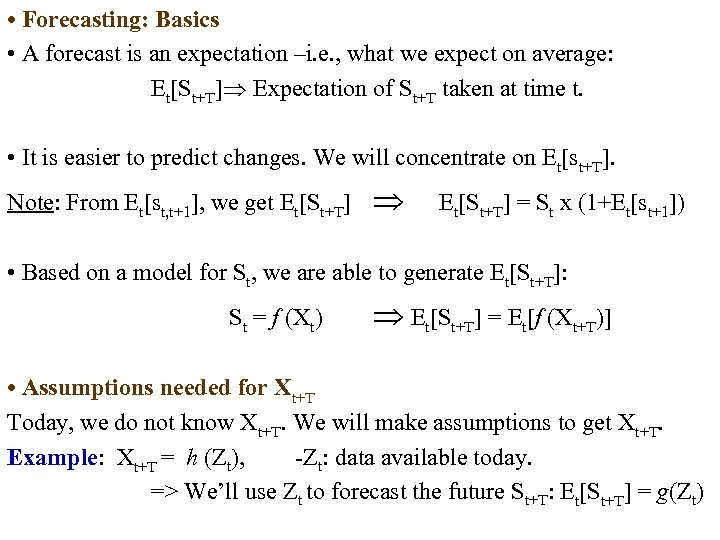• Forecasting: Basics • A forecast is an expectation –i. e. , what we expect on average: Et[St+T] Expectation of St+T taken at time t. • It is easier to predict changes. We will concentrate on Et[st+T]. Note: From Et[st, t+1], we get Et[St+T] = St x (1+Et[st+1]) • Based on a model for St, we are able to generate Et[St+T]: St = f (Xt) Et[St+T] = Et[f (Xt+T)] • Assumptions needed for Xt+T Today, we do not know Xt+T. We will make assumptions to get Xt+T. Example: Xt+T = h (Zt), -Zt: data available today. => We’ll use Zt to forecast the future St+T: Et[St+T] = g(Zt)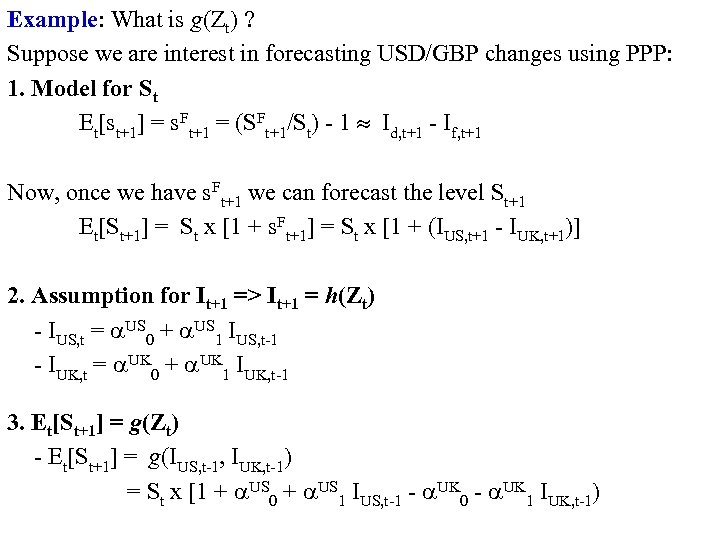Example: What is g(Zt) ? Suppose we are interest in forecasting USD/GBP changes using PPP: 1. Model for St Et[st+1] = s. Ft+1 = (SFt+1/St) - 1 Id, t+1 - If, t+1 Now, once we have s. Ft+1 we can forecast the level St+1 Et[St+1] = St x [1 + s. Ft+1] = St x [1 + (IUS, t+1 - IUK, t+1)] 2. Assumption for It+1 => It+1 = h(Zt) - IUS, t = US 0 + US 1 IUS, t-1 - IUK, t = UK 0 + UK 1 IUK, t-1 3. Et[St+1] = g(Zt) - Et[St+1] = g(IUS, t-1, IUK, t-1) = St x [1 + US 0 + US 1 IUS, t-1 - UK 0 - UK 1 IUK, t-1)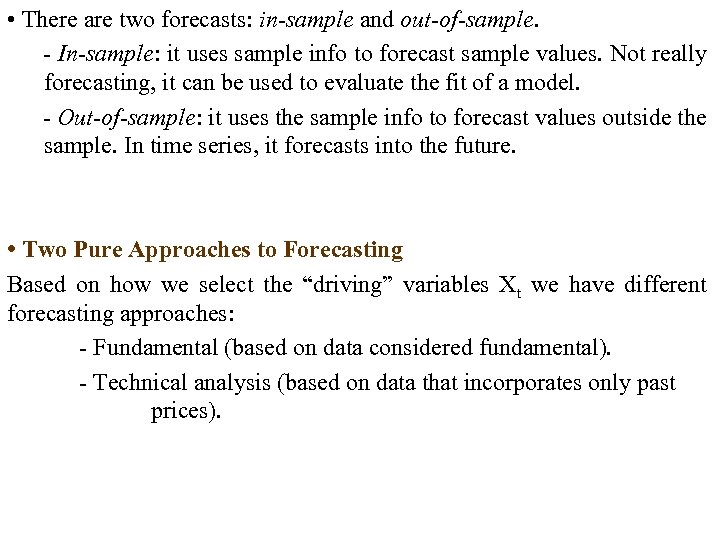• There are two forecasts: in-sample and out-of-sample. - In-sample: it uses sample info to forecast sample values. Not really forecasting, it can be used to evaluate the fit of a model. - Out-of-sample: it uses the sample info to forecast values outside the sample. In time series, it forecasts into the future. • Two Pure Approaches to Forecasting Based on how we select the “driving” variables Xt we have different forecasting approaches: - Fundamental (based on data considered fundamental). - Technical analysis (based on data that incorporates only past prices).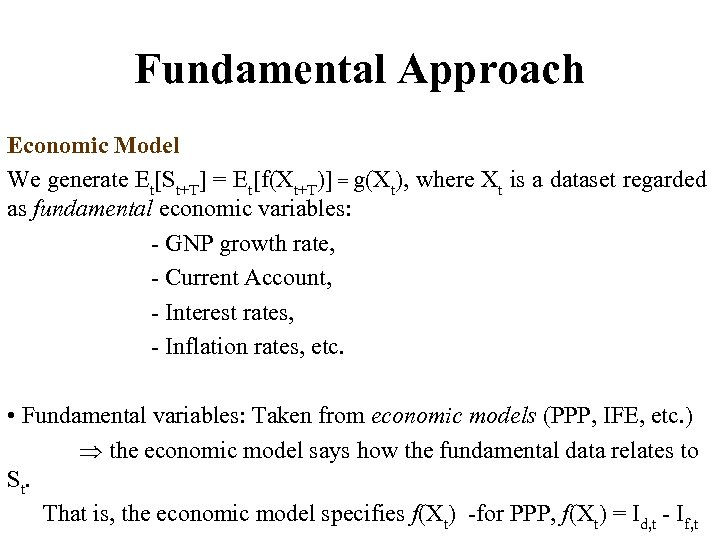Fundamental Approach Economic Model We generate Et[St+T] = Et[f(Xt+T)] = g(Xt), where Xt is a dataset regarded as fundamental economic variables: - GNP growth rate, - Current Account, - Interest rates, - Inflation rates, etc. • Fundamental variables: Taken from economic models (PPP, IFE, etc. ) the economic model says how the fundamental data relates to St. That is, the economic model specifies f(Xt) -for PPP, f(Xt) = Id, t - If, t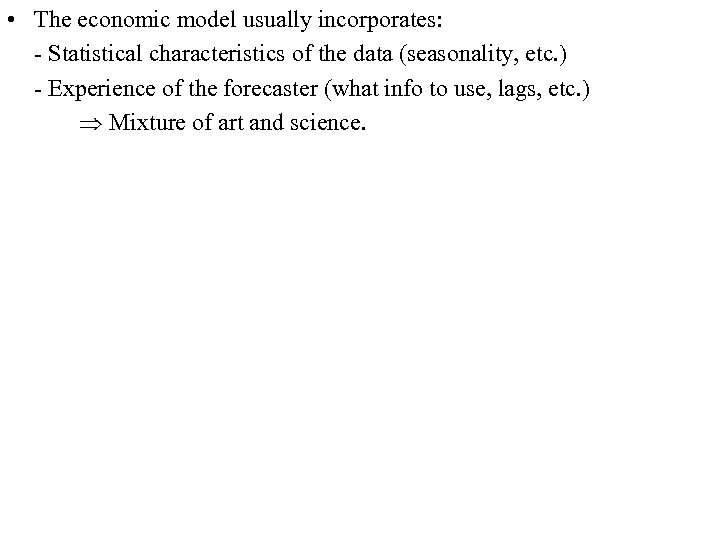• The economic model usually incorporates: - Statistical characteristics of the data (seasonality, etc. ) - Experience of the forecaster (what info to use, lags, etc. ) Mixture of art and science.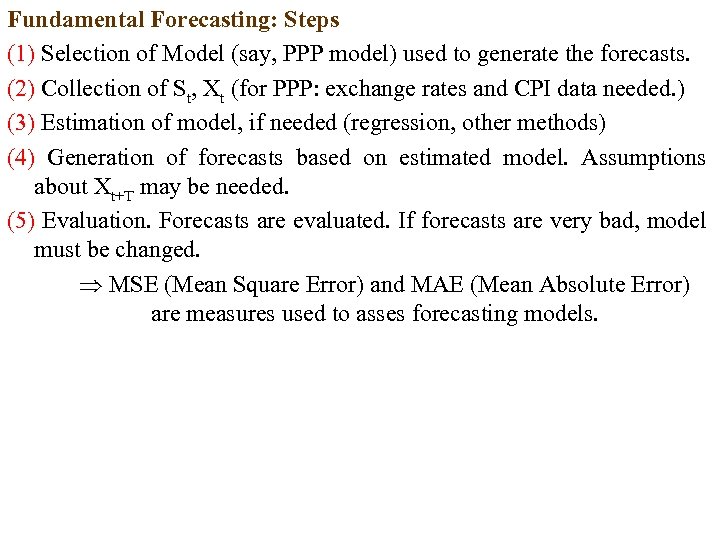Fundamental Forecasting: Steps (1) Selection of Model (say, PPP model) used to generate the forecasts. (2) Collection of St, Xt (for PPP: exchange rates and CPI data needed. ) (3) Estimation of model, if needed (regression, other methods) (4) Generation of forecasts based on estimated model. Assumptions about Xt+T may be needed. (5) Evaluation. Forecasts are evaluated. If forecasts are very bad, model must be changed. MSE (Mean Square Error) and MAE (Mean Absolute Error) are measures used to asses forecasting models.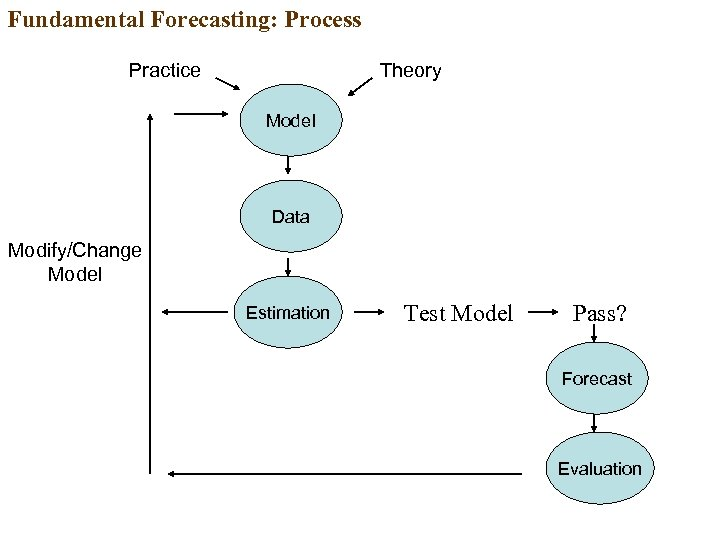Fundamental Forecasting: Process Practice Theory Model Data Modify/Change Model Estimation Test Model Pass? Forecast Evaluation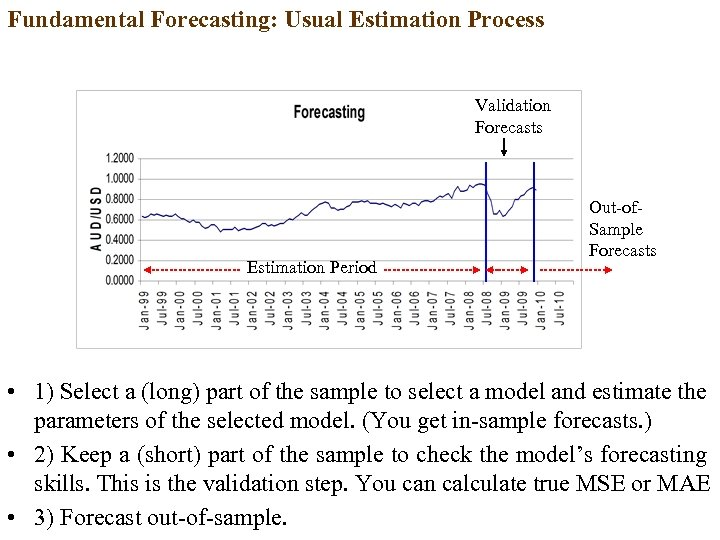Fundamental Forecasting: Usual Estimation Process Validation Forecasts Estimation Period Out-of. Sample Forecasts • 1) Select a (long) part of the sample to select a model and estimate the parameters of the selected model. (You get in-sample forecasts. ) • 2) Keep a (short) part of the sample to check the model’s forecasting skills. This is the validation step. You can calculate true MSE or MAE • 3) Forecast out-of-sample.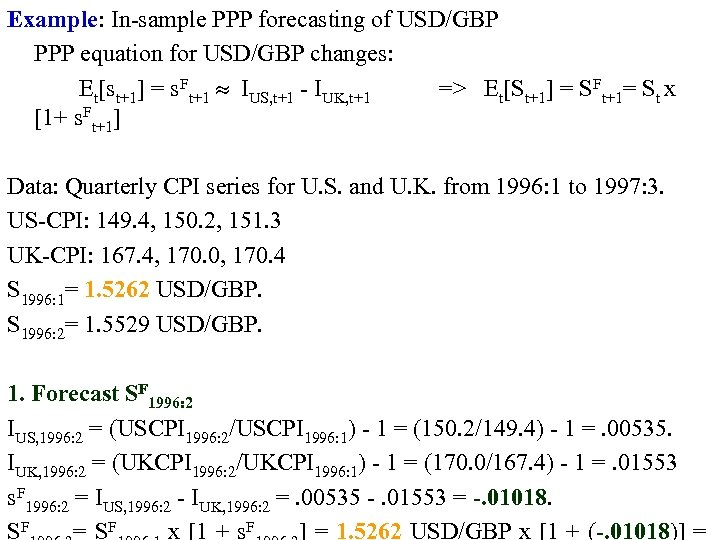Example: In-sample PPP forecasting of USD/GBP PPP equation for USD/GBP changes: Et[st+1] = s. Ft+1 IUS, t+1 - IUK, t+1 => Et[St+1] = SFt+1= St x [1+ s. Ft+1] Data: Quarterly CPI series for U. S. and U. K. from 1996: 1 to 1997: 3. US-CPI: 149. 4, 150. 2, 151. 3 UK-CPI: 167. 4, 170. 0, 170. 4 S 1996: 1= 1. 5262 USD/GBP. S 1996: 2= 1. 5529 USD/GBP. 1. Forecast SF 1996: 2 IUS, 1996: 2 = (USCPI 1996: 2/USCPI 1996: 1) - 1 = (150. 2/149. 4) - 1 =. 00535. IUK, 1996: 2 = (UKCPI 1996: 2/UKCPI 1996: 1) - 1 = (170. 0/167. 4) - 1 =. 01553 s. F 1996: 2 = IUS, 1996: 2 - IUK, 1996: 2 =. 00535 -. 01553 = -. 01018. SF = SF x [1 + s. F ] = 1. 5262 USD/GBP x [1 + (-. 01018)] =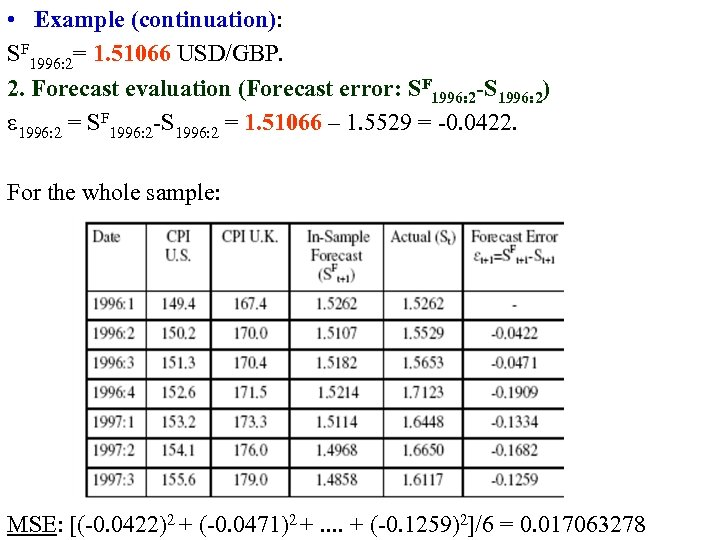• Example (continuation): SF 1996: 2= 1. 51066 USD/GBP. 2. Forecast evaluation (Forecast error: SF 1996: 2 -S 1996: 2) 1996: 2 = SF 1996: 2 -S 1996: 2 = 1. 51066 – 1. 5529 = -0. 0422. For the whole sample: MSE: [(-0. 0422)2 + (-0. 0471)2 +. . + (-0. 1259)2]/6 = 0. 017063278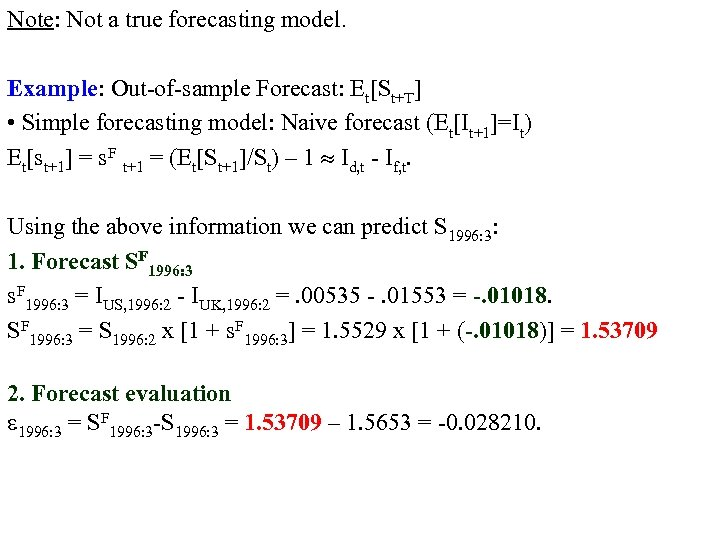Note: Not a true forecasting model. Example: Out-of-sample Forecast: Et[St+T] • Simple forecasting model: Naive forecast (Et[It+1]=It) Et[st+1] = s. F t+1 = (Et[St+1]/St) – 1 Id, t - If, t. Using the above information we can predict S 1996: 3: 1. Forecast SF 1996: 3 s. F 1996: 3 = IUS, 1996: 2 - IUK, 1996: 2 =. 00535 -. 01553 = -. 01018. SF 1996: 3 = S 1996: 2 x [1 + s. F 1996: 3] = 1. 5529 x [1 + (-. 01018)] = 1. 53709 2. Forecast evaluation 1996: 3 = SF 1996: 3 -S 1996: 3 = 1. 53709 – 1. 5653 = -0. 028210.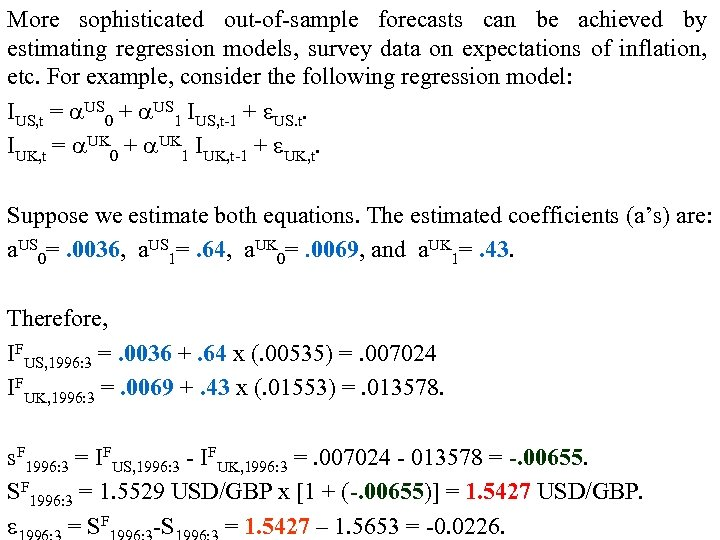More sophisticated out-of-sample forecasts can be achieved by estimating regression models, survey data on expectations of inflation, etc. For example, consider the following regression model: IUS, t = US 0 + US 1 IUS, t-1 + US. t. IUK, t = UK 0 + UK 1 IUK, t-1 + UK, t. Suppose we estimate both equations. The estimated coefficients (a’s) are: a. US 0=. 0036, a. US 1=. 64, a. UK 0=. 0069, and a. UK 1=. 43. Therefore, IFUS, 1996: 3 =. 0036 +. 64 x (. 00535) =. 007024 IFUK, 1996: 3 =. 0069 +. 43 x (. 01553) =. 013578. s. F 1996: 3 = IFUS, 1996: 3 - IFUK, 1996: 3 =. 007024 - 013578 = -. 00655. SF 1996: 3 = 1. 5529 USD/GBP x [1 + (-. 00655)] = 1. 5427 USD/GBP. = SF -S = 1. 5427 – 1. 5653 = -0. 0226.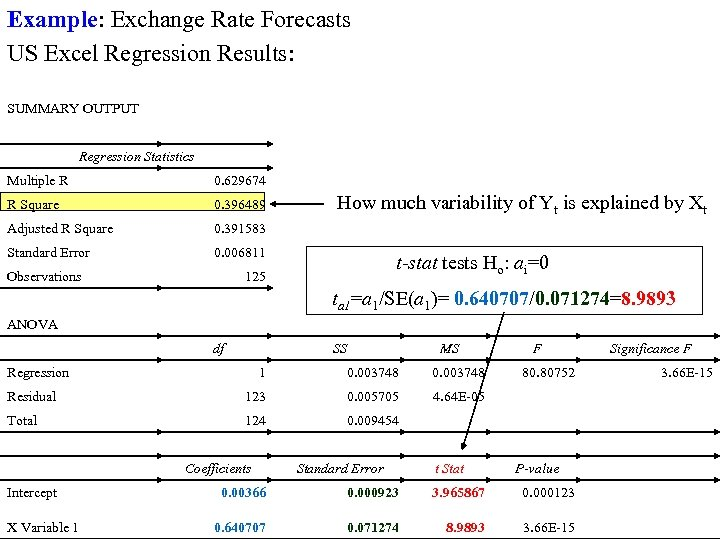Example: Exchange Rate Forecasts US Excel Regression Results: SUMMARY OUTPUT Regression Statistics Multiple R 0. 629674 R Square 0. 396489 Adjusted R Square 0. 391583 Standard Error 0. 006811 Observations How much variability of Yt is explained by Xt t-stat tests Ho: ai=0 125 ta 1=a 1/SE(a 1)= 0. 640707/0. 071274=8. 9893 ANOVA df SS Regression MS 1 0. 003748 Residual 123 0. 005705 124 0. 009454 Significance F 4. 64 E-05 Total F Intercept X Variable 1 Coefficients Standard Error 80. 80752 t Stat 3. 66 E-15 P-value 0. 00366 0. 000923 3. 965867 0. 000123 0. 640707 0. 071274 8. 9893 3. 66 E-15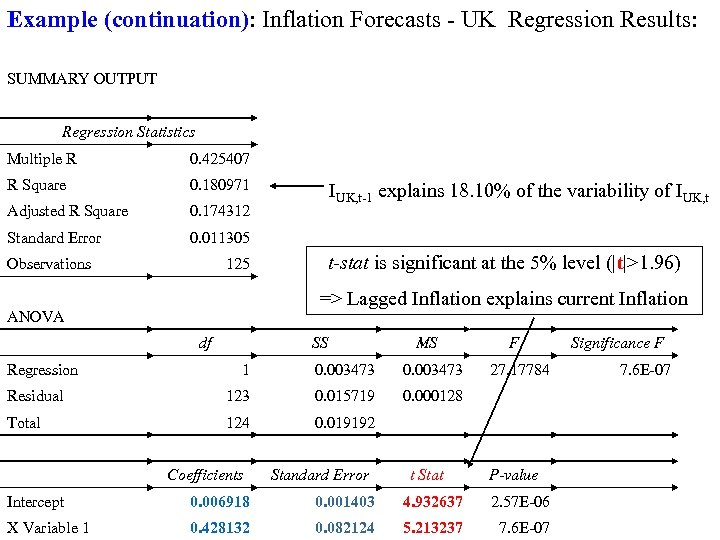Example (continuation): Inflation Forecasts - UK Regression Results: SUMMARY OUTPUT Regression Statistics Multiple R 0. 425407 R Square 0. 180971 Adjusted R Square 0. 174312 Standard Error 0. 011305 Observations 125 IUK, t-1 explains 18. 10% of the variability of IUK, t t-stat is significant at the 5% level (|t|>1. 96) => Lagged Inflation explains current Inflation ANOVA Regression df SS MS 1 0. 003473 Residual 123 0. 015719 124 0. 019192 Significance F 0. 000128 Total F Coefficients Standard Error 27. 17784 t Stat Intercept 0. 006918 0. 001403 4. 932637 X Variable 1 0. 428132 0. 082124 5. 213237 7. 6 E-07 P-value 2. 57 E-06 7. 6 E-07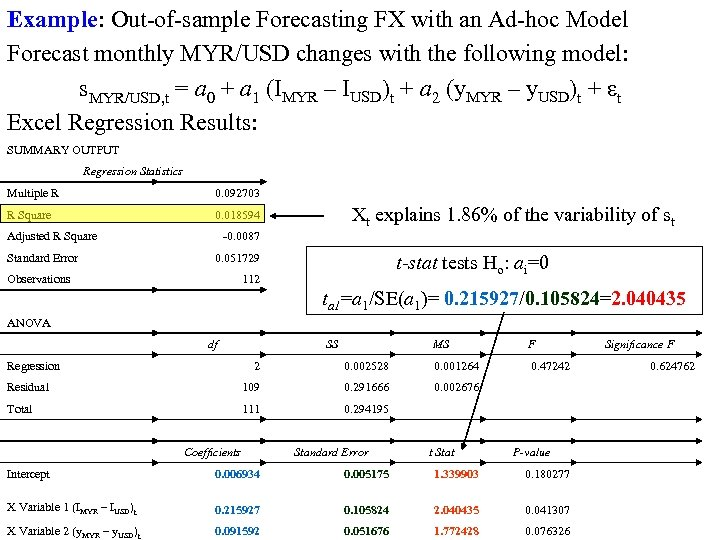Example: Out-of-sample Forecasting FX with an Ad-hoc Model Forecast monthly MYR/USD changes with the following model: s. MYR/USD, t = a 0 + a 1 (IMYR – IUSD)t + a 2 (y. MYR – y. USD)t + εt Excel Regression Results: SUMMARY OUTPUT Regression Statistics Multiple R 0. 092703 R Square 0. 018594 Adjusted R Square Xt explains 1. 86% of the variability of st -0. 0087 Standard Error t-stat tests Ho: ai=0 0. 051729 Observations 112 ta 1=a 1/SE(a 1)= 0. 215927/0. 105824=2. 040435 ANOVA df Regression SS MS F 0. 47242 2 0. 002528 0. 001264 Residual 109 0. 291666 0. 002676 Total 111 0. 294195 Significance F Coefficients Intercept Standard Error t Stat 0. 624762 P-value 0. 006934 0. 005175 1. 339903 0. 180277 0. 215927 0. 105824 2. 040435 0. 041307 X Variable 2 (y. MYR – y. USD)t 0. 091592 0. 051676 1. 772428 0. 076326 X Variable 1 (IMYR – IUSD)t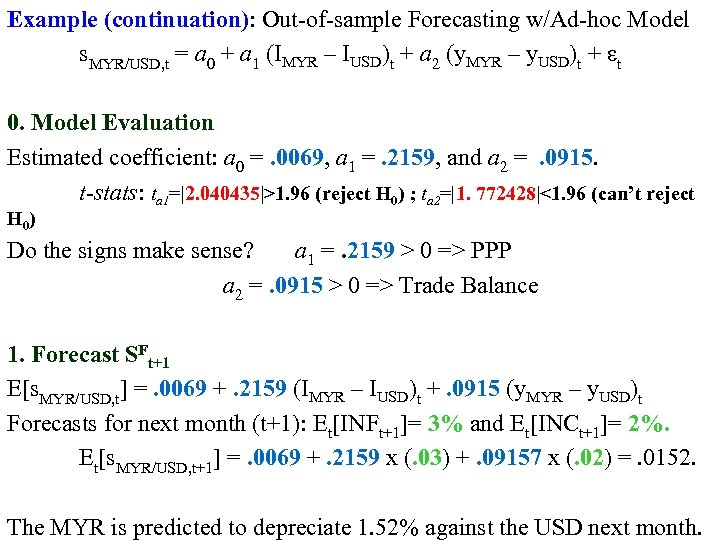Example (continuation): Out-of-sample Forecasting w/Ad-hoc Model s. MYR/USD, t = a 0 + a 1 (IMYR – IUSD)t + a 2 (y. MYR – y. USD)t + εt 0. Model Evaluation Estimated coefficient: a 0 =. 0069, a 1 =. 2159, and a 2 = . 0915. t-stats: ta 1=|2. 040435|>1. 96 (reject H 0) ; ta 2=|1. 772428|<1. 96 (can’t reject H 0 ) Do the signs make sense? a 1 =. 2159 > 0 => PPP a 2 =. 0915 > 0 => Trade Balance 1. Forecast SFt+1 E[s. MYR/USD, t] =. 0069 +. 2159 (IMYR – IUSD)t +. 0915 (y. MYR – y. USD)t Forecasts for next month (t+1): Et[INFt+1]= 3% and Et[INCt+1]= 2%. Et[s. MYR/USD, t+1] =. 0069 +. 2159 x (. 03) +. 09157 x (. 02) =. 0152. The MYR is predicted to depreciate 1. 52% against the USD next month.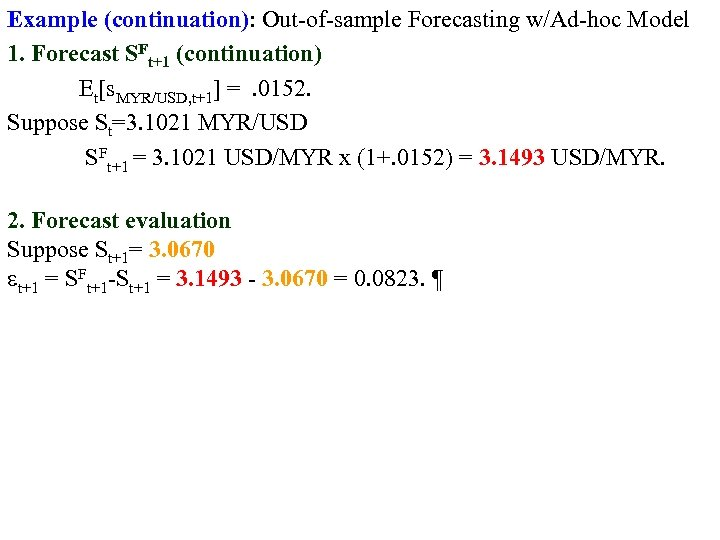Example (continuation): Out-of-sample Forecasting w/Ad-hoc Model 1. Forecast SFt+1 (continuation) Et[s. MYR/USD, t+1] = . 0152. Suppose St=3. 1021 MYR/USD SFt+1 = 3. 1021 USD/MYR x (1+. 0152) = 3. 1493 USD/MYR. 2. Forecast evaluation Suppose St+1= 3. 0670 t+1 = SFt+1 -St+1 = 3. 1493 - 3. 0670 = 0. 0823. ¶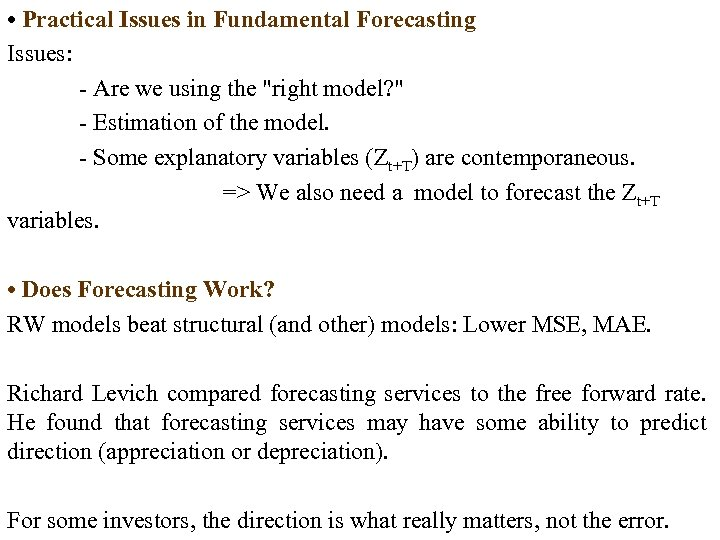• Practical Issues in Fundamental Forecasting Issues: - Are we using the "right model? " - Estimation of the model. - Some explanatory variables (Zt+T) are contemporaneous. => We also need a model to forecast the Zt+T variables. • Does Forecasting Work? RW models beat structural (and other) models: Lower MSE, MAE. Richard Levich compared forecasting services to the free forward rate. He found that forecasting services may have some ability to predict direction (appreciation or depreciation). For some investors, the direction is what really matters, not the error.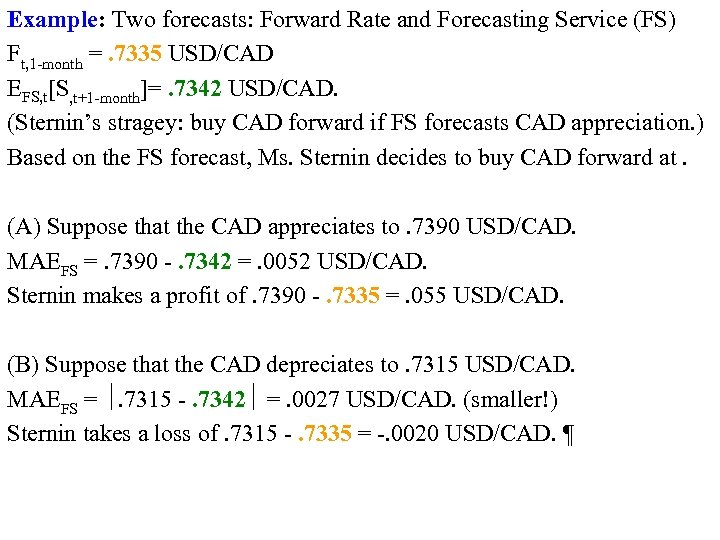Example: Two forecasts: Forward Rate and Forecasting Service (FS) Ft, 1 -month =. 7335 USD/CAD EFS, t[S, t+1 -month]=. 7342 USD/CAD. (Sternin’s stragey: buy CAD forward if FS forecasts CAD appreciation. ) Based on the FS forecast, Ms. Sternin decides to buy CAD forward at. (A) Suppose that the CAD appreciates to. 7390 USD/CAD. MAEFS =. 7390 -. 7342 =. 0052 USD/CAD. Sternin makes a profit of. 7390 -. 7335 =. 055 USD/CAD. (B) Suppose that the CAD depreciates to. 7315 USD/CAD. MAEFS = . 7315 -. 7342 =. 0027 USD/CAD. (smaller!) Sternin takes a loss of. 7315 -. 7335 = -. 0020 USD/CAD. ¶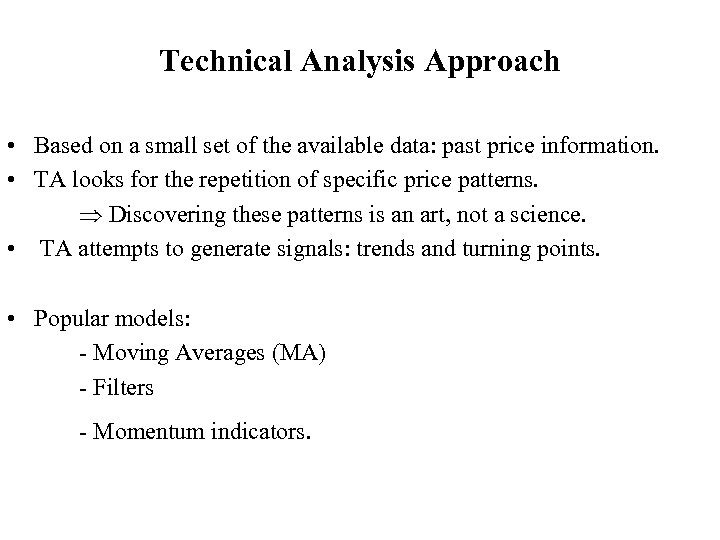Technical Analysis Approach • Based on a small set of the available data: past price information. • TA looks for the repetition of specific price patterns. Discovering these patterns is an art, not a science. • TA attempts to generate signals: trends and turning points. • Popular models: - Moving Averages (MA) - Filters - Momentum indicators.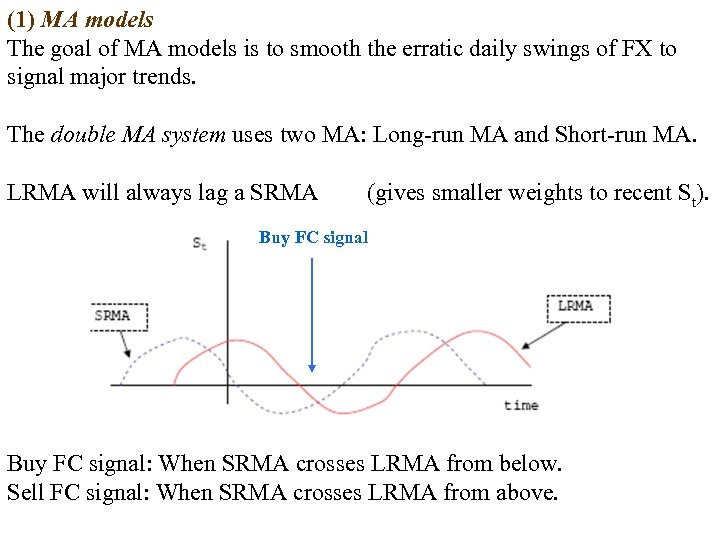(1) MA models The goal of MA models is to smooth the erratic daily swings of FX to signal major trends. The double MA system uses two MA: Long-run MA and Short-run MA. LRMA will always lag a SRMA (gives smaller weights to recent St). Buy FC signal: When SRMA crosses LRMA from below. Sell FC signal: When SRMA crosses LRMA from above.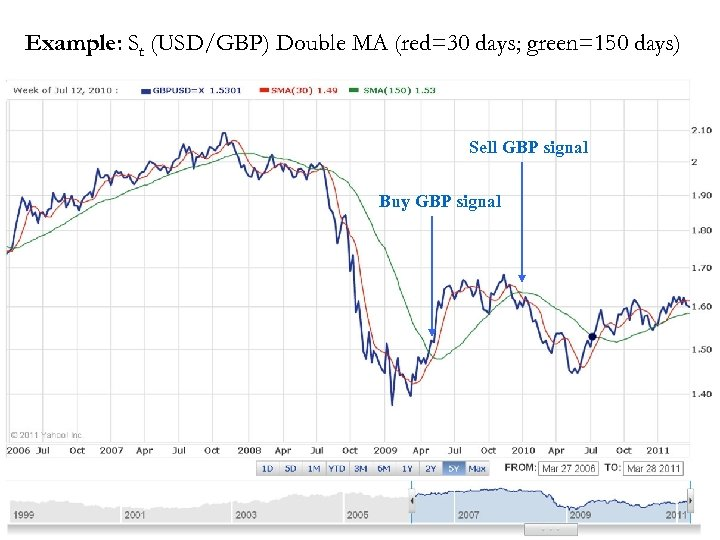Example: St (USD/GBP) Double MA (red=30 days; green=150 days) Sell GBP signal Buy GBP signal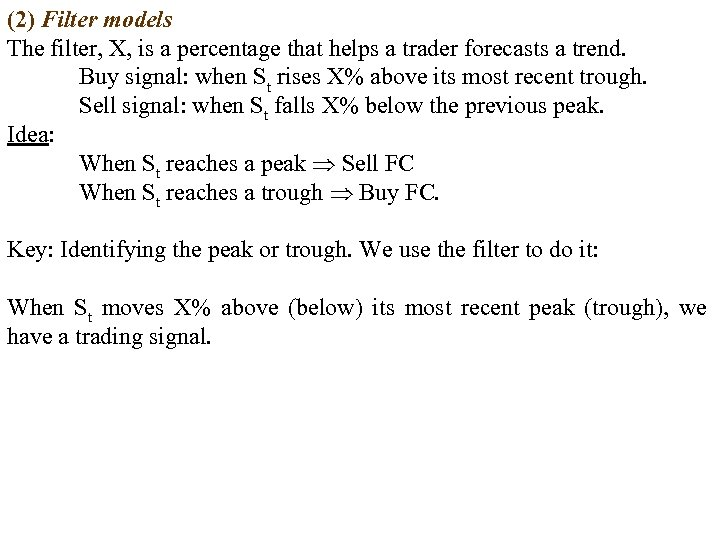(2) Filter models The filter, X, is a percentage that helps a trader forecasts a trend. Buy signal: when St rises X% above its most recent trough. Sell signal: when St falls X% below the previous peak. Idea: When St reaches a peak Sell FC When St reaches a trough Buy FC. Key: Identifying the peak or trough. We use the filter to do it: When St moves X% above (below) its most recent peak (trough), we have a trading signal.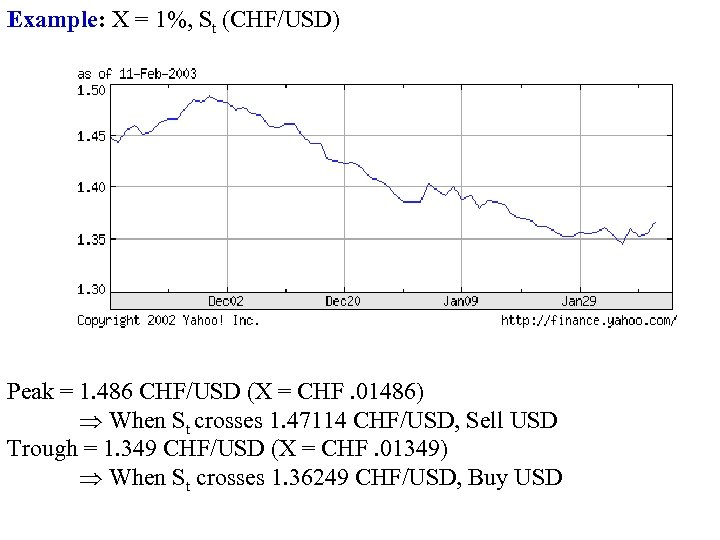Example: X = 1%, St (CHF/USD) Peak = 1. 486 CHF/USD (X = CHF. 01486) When St crosses 1. 47114 CHF/USD, Sell USD Trough = 1. 349 CHF/USD (X = CHF. 01349) When St crosses 1. 36249 CHF/USD, Buy USD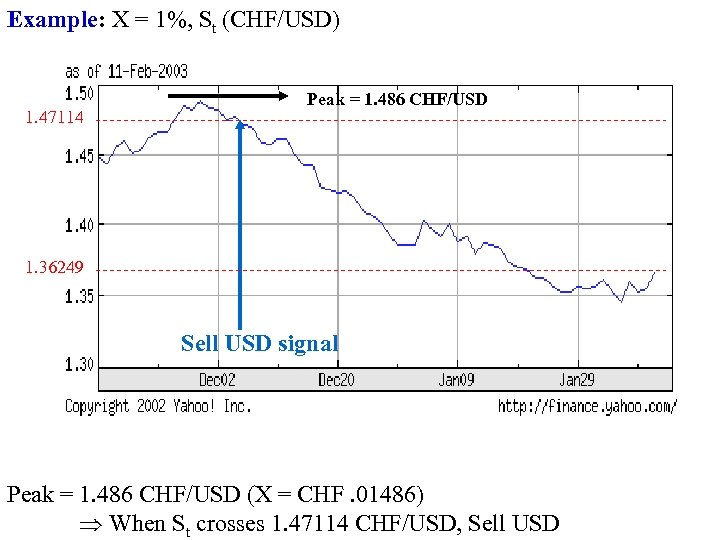Example: X = 1%, St (CHF/USD) 1. 47114 Peak = 1. 486 CHF/USD 1. 36249 Sell USD signal Peak = 1. 486 CHF/USD (X = CHF. 01486) When St crosses 1. 47114 CHF/USD, Sell USD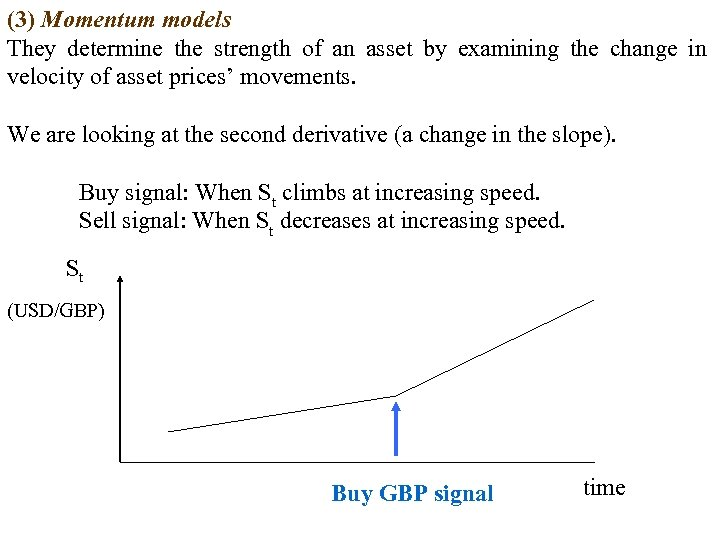(3) Momentum models They determine the strength of an asset by examining the change in velocity of asset prices’ movements. We are looking at the second derivative (a change in the slope). Buy signal: When St climbs at increasing speed. Sell signal: When St decreases at increasing speed. St (USD/GBP) Buy GBP signal time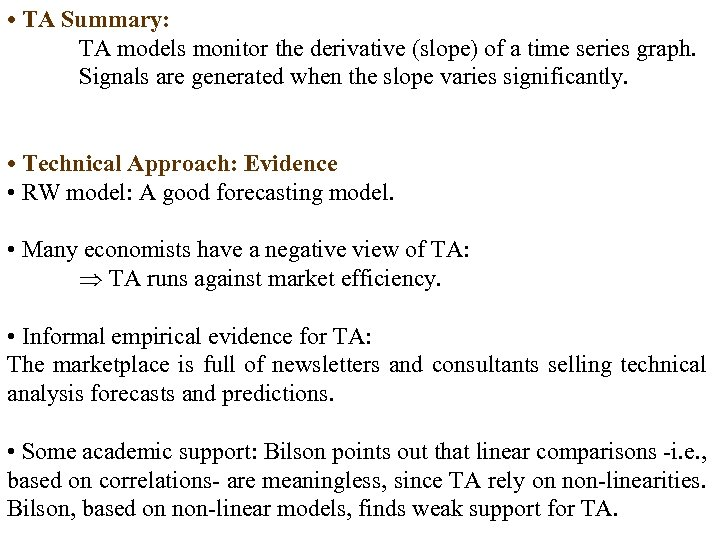• TA Summary: TA models monitor the derivative (slope) of a time series graph. Signals are generated when the slope varies significantly. • Technical Approach: Evidence • RW model: A good forecasting model. • Many economists have a negative view of TA: TA runs against market efficiency. • Informal empirical evidence for TA: The marketplace is full of newsletters and consultants selling technical analysis forecasts and predictions. • Some academic support: Bilson points out that linear comparisons -i. e. , based on correlations- are meaningless, since TA rely on non-linearities. Bilson, based on non-linear models, finds weak support for TA.